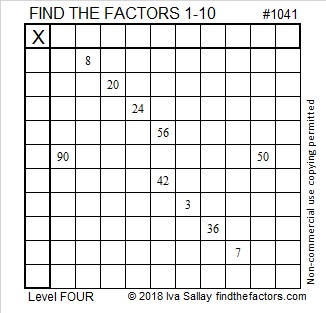# 1041 and Level 4

Any level 3 puzzle can be easily made into a level 4 puzzle by removing some restrictions on the order of the clues. If you can solve a level 3 puzzle, then this level 4 puzzle will be only a little more difficult to solve.Print the puzzles or type the solution in this excel file: 10-factors-1035-1043

What have I found out about the number 1041?

• 1041 is a composite number.
• Prime factorization: 1041 = 3 × 347
• The exponents in the prime factorization are 1 and 1. Adding one to each and multiplying we get (1 + 1)(1 + 1) = 2 × 2 = 4. Therefore 1041 has exactly 4 factors.
• Factors of 1041: 1, 3, 347, 1041
• Factor pairs: 1041 = 1 × 1041 or 3 × 347
• 1041 has no square factors that allow its square root to be simplified. √1041 ≈ 32.264531041 is also a palindrome in three other bases:
It’s 13131 in BASE 5 because 5⁴ + 3(5³) + 5² +3(5) + 1 = 1041,
545 in BASE 14 because 5(14²) + 4(14) + 5(1) = 1041, and
1E1 in BASE 26 (E is 14 base 10) because 26² + 14(26) + 1 = 1041

This site uses Akismet to reduce spam. Learn how your comment data is processed.